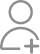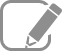Take Class 11 Tuition from the Best Tutors

•Affordable fees
•1-1 or Group class
•Flexible Timings
•Verified Tutors

Search in

# (a) What is the largest average velocity of blood flow in an artery of radius 2 × 10–3 m if the flow must remain laminar? (b) What is the corresponding flow rate? (Take viscosity of blood to be 2.084 × 10–3 Pa s).Follow 1Answer(a)Radius of the artery, r = 2 × 10–3 m Diameter of the artery, d = 2 × 2 × 10–3 m = 4 × 10– 3 m Viscosity of blood, Density of blood, ρ = 1.06 × 103 kg/m3 Reynolds’ number for laminar flow, NR = 2000 The largest average velocity of blood is...

(a)Radius of the artery, r = 2 × 10–3 m

Diameter of the artery, d = 2 × 2 × 10–3 m = 4 × 10– 3 m

Viscosity of blood,Density of blood, ρ = 1.06 × 103 kg/m3

Reynolds’ number for laminar flow, NR = 2000

The largest average velocity of blood is given by the relation:Therefore, the largest average velocity of blood is 0.983 m/s.

(b) Flow rate is given by the relation:

R = π r2Therefore, the corresponding flow rate is.

Now ask question in any of the 1000+ Categories, and get Answers from Tutors and Trainers on UrbanPro.com

Find Grade 11 Tuition near you

Looking for Class 11 Tuition ?

Learn from the Best Tutors on UrbanPro

Are you a Tutor or Training Institute?

Join UrbanPro Today to find students near you
X

### Looking for Class 11 Tuition Classes?

The best tutors for Class 11 Tuition Classes are on UrbanPro

• Select the best Tutor
• Book & Attend a Free Demo
• Pay and start Learning### Take Class 11 Tuition with the Best Tutors

The best Tutors for Class 11 Tuition Classes are on UrbanPro